# Count ways to change direction of edges such that graph becomes acyclic

Given a directed and unweighted graph consisting of N vertices and an array arr[] where ith vertex have a directed edge to arr[i]. The task is to find the number of ways to change the direction of edges such that the given graph is acyclic.

Examples:

Input: N = 3, arr[] = {2, 3, 1}
The directed graph form by the given infromation is: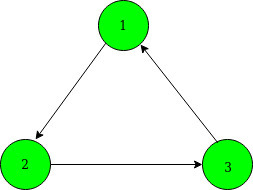Output: 6
Explanation:
There are 6 possible ways to change the direction of edges to make grpah acyclic: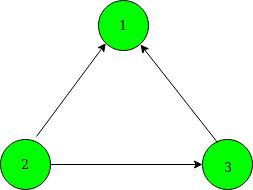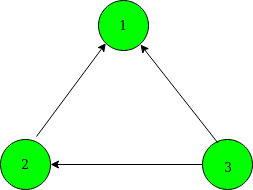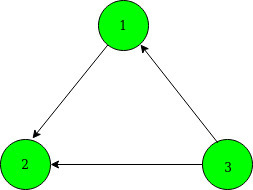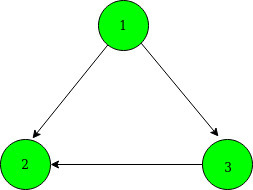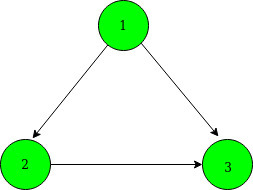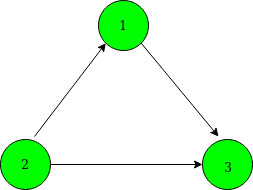## Recommended: Please try your approach on {IDE} first, before moving on to the solution.

Approach: The idea is to check whether the Connected Components form a cycle or not.

• If the component is a path, then however we orient the edges we won’t form a cycle.
• If the component has a cycle with N edges, then there are 2N ways to arrange all the edges out of which only 2 ways are going to form a cycle. So there are (2N – 2) ways to change the edges so that graph becomes acyclic.

Steps:

1. Using Depth First Search(DFS) traversal find the cycles in the given graph and number of vertices associated with each cycle.
2. After DFS traversal, the total number of ways to change the direction of edges is the product of the following:
• Number of ways form by each cycle of X vertices is given by (2X – 2).
• Number of ways form by each path of Y vertices is given by (2Y).

Below is the implementation of the above approach:

## C++

 `// C++ program to count the ` `// number of ways to change ` `// the direction of edges ` `// such that no cycle is ` `// present in the graph ` `#include ` `using` `namespace` `std; ` ` `  `// Vector cycles[] to store ` `// the cycle with vertices ` `// associated with each cycle ` `vector<``int``> cycles; ` ` `  `// Count of cycle ` `int` `cyclecnt; ` ` `  `// Function to count the ` `// number of vertices in the ` `// current cycle ` `void` `DFSUtil(``int` `u, ``int` `arr[], ``int` `vis[]) ` `{ ` `    ``cycles[cyclecnt]++; ` `    ``vis[u] = 3; ` ` `  `    ``// Returns when the same ` `    ``// initial vertex is found ` `    ``if` `(vis[arr[u]] == 3) { ` `        ``return``; ` `    ``} ` ` `  `    ``// Recurr for next vertex ` `    ``DFSUtil(arr[u], arr, vis); ` `} ` ` `  `// DFS traversal to detect ` `// the cycle in graph ` `void` `DFS(``int` `u, ``int` `arr[], ``int` `vis[]) ` `{ ` `    ``// Marke vis[u] to 2 to ` `    ``// check for any cycle form ` `    ``vis[u] = 2; ` ` `  `    ``// If the vertex arr[u] ` `    ``// is not visited ` `    ``if` `(vis[arr[u]] == 0) { ` `        ``// Call DFS ` `        ``DFS(arr[u], arr, vis); ` `    ``} ` ` `  `    ``// If current node is ` `    ``// processed ` `    ``else` `if` `(vis[arr[u]] == 1) { ` `        ``vis[u] = 1; ` `        ``return``; ` `    ``} ` ` `  `    ``// Cycle found, call DFSUtil ` `    ``// to count the number of ` `    ``// vertices in the current ` `    ``// cycle ` `    ``else` `{ ` `        ``cycles.push_back(0); ` ` `  `        ``// Count number of ` `        ``// vertices in cycle ` `        ``DFSUtil(u, arr, vis); ` `        ``cyclecnt++; ` `    ``} ` ` `  `    ``// Current Node is processed ` `    ``vis[u] = 1; ` `} ` ` `  `// Function to count the ` `// number of ways ` `int` `countWays(``int` `arr[], ``int` `N) ` `{ ` ` `  `    ``int` `i, ans = 1; ` ` `  `    ``// To precompute the power ` `    ``// of 2 ` `    ``int` `dp[N + 1]; ` `    ``dp = 1; ` ` `  `    ``// Storing power of 2 ` `    ``for` `(``int` `i = 1; i <= N; i++) { ` `        ``dp[i] = (dp[i - 1] * 2); ` `    ``} ` ` `  `    ``// Array vis[] created for ` `    ``// DFS traversal ` `    ``int` `vis[N + 1] = { 0 }; ` ` `  `    ``// DFS traversal from Node 1 ` `    ``for` `(``int` `i = 1; i <= N; i++) { ` `        ``if` `(vis[i] == 0) { ` ` `  `            ``// Calling DFS ` `            ``DFS(i, arr, vis); ` `        ``} ` `    ``} ` ` `  `    ``int` `cnt = N; ` ` `  `    ``// Traverse the cycles array ` `    ``for` `(i = 0; i < cycles.size(); i++) { ` ` `  `        ``// Remove the vertices ` `        ``// which are part of a ` `        ``// cycle ` `        ``cnt -= cycles[i]; ` ` `  `        ``// Count form by number ` `        ``// vertices form cycle ` `        ``ans *= dp[cycles[i]] - 2; ` `    ``} ` ` `  `    ``// Count form by number of ` `    ``// vertices not forming ` `    ``// cycle ` `    ``ans = (ans * dp[cnt]); ` ` `  `    ``return` `ans; ` `} ` ` `  `// Driver's Code ` `int` `main() ` `{ ` `    ``int` `N = 3; ` `    ``int` `arr[] = { 0, 2, 3, 1 }; ` ` `  `    ``// Function to count ways ` `    ``cout << countWays(arr, N); ` `    ``return` `0; ` `} `

## Python3

 `# Python 3 program to count the ` `# number of ways to change ` `# the direction of edges ` `# such that no cycle is ` `# present in the graph ` ` `  `# List cycles[] to store ` `# the cycle with vertices ` `# associated with each cycle ` `cycles ``=` `[] ` ` `  `# Function to count the ` `# number of vertices in the ` `# current cycle ` `def` `DFSUtil(u, arr, vis, cyclecnt): ` ` `  `    ``cycles[cyclecnt] ``+``=` `1` `    ``vis[u] ``=` `3` ` `  `    ``# Returns when the same ` `    ``# initial vertex is found ` `    ``if` `(vis[arr[u]] ``=``=` `3``) : ` `        ``return` ` `  `    ``# Recurr for next vertex ` `    ``DFSUtil(arr[u], arr, vis, cyclecnt) ` ` `  `# DFS traversal to detect ` `# the cycle in graph ` `def` `DFS( u, arr, vis, cyclecnt): ` ` `  `    ``# Marke vis[u] to 2 to ` `    ``# check for any cycle form ` `    ``vis[u] ``=` `2` ` `  `    ``# If the vertex arr[u] ` `    ``# is not visited ` `    ``if` `(vis[arr[u]] ``=``=` `0``) : ` `         `  `        ``# Call DFS ` `        ``DFS(arr[u], arr, vis, cyclecnt) ` ` `  `    ``# If current node is ` `    ``# processed ` `    ``elif` `(vis[arr[u]] ``=``=` `1``): ` `        ``vis[u] ``=` `1` `        ``return` ` `  `    ``# Cycle found, call DFSUtil ` `    ``# to count the number of ` `    ``# vertices in the current ` `    ``# cycle ` `    ``else` `: ` `        ``cycles.append(``0``) ` ` `  `        ``# Count number of ` `        ``# vertices in cycle ` `        ``DFSUtil(u, arr, vis,cyclecnt) ` `        ``cyclecnt ``+``=` `1` ` `  `    ``# Current Node is processed ` `    ``vis[u] ``=` `1` ` `  `# Function to count the ` `# number of ways ` `def` `countWays(arr, N,cyclecnt): ` ` `  `    ``ans ``=` `1` ` `  `    ``# To precompute the power ` `    ``# of 2 ` `    ``dp ``=` `[``0``]``*``(N ``+` `1``) ` `    ``dp[``0``] ``=` `1` ` `  `    ``# Storing power of 2 ` `    ``for` `i ``in` `range``(``1``, N ``+` `1``): ` `        ``dp[i] ``=` `(dp[i ``-` `1``] ``*` `2``) ` ` `  `    ``# Array vis[] created for ` `    ``# DFS traversal ` `    ``vis ``=` `[``0``]``*``(N ``+` `1``) ` ` `  `    ``# DFS traversal from Node 1 ` `    ``for` `i ``in` `range``(``1``, N ``+` `1``) : ` `        ``if` `(vis[i] ``=``=` `0``) : ` ` `  `            ``# Calling DFS ` `            ``DFS(i, arr, vis, cyclecnt) ` ` `  `    ``cnt ``=` `N ` ` `  `    ``# Traverse the cycles array ` `    ``for` `i ``in` `range``(``len``(cycles)) : ` ` `  `        ``# Remove the vertices ` `        ``# which are part of a ` `        ``# cycle ` `        ``cnt ``-``=` `cycles[i] ` ` `  `        ``# Count form by number ` `        ``# vertices form cycle ` `        ``ans ``*``=` `dp[cycles[i]] ``-` `2` ` `  `    ``# Count form by number of ` `    ``# vertices not forming ` `    ``# cycle ` `    ``ans ``=` `(ans ``*` `dp[cnt]) ` ` `  `    ``return` `ans ` ` `  `# Driver's Code ` `if` `__name__ ``=``=` `"__main__"``: ` `     `  `    ``N ``=` `3` `    ``cyclecnt ``=` `0` `    ``arr ``=` `[ ``0``, ``2``, ``3``, ``1` `] ` ` `  `    ``# Function to count ways ` `    ``print``(countWays(arr, N,cyclecnt)) ` `     `  `# This code is contributed by chitranayal `

Output:

```6
```

Time Complexity : O(V + E)

Attention reader! Don’t stop learning now. Get hold of all the important DSA concepts with the DSA Self Paced Course at a student-friendly price and become industry ready.

My Personal Notes arrow_drop_upCheck out this Author's contributed articles.

If you like GeeksforGeeks and would like to contribute, you can also write an article using contribute.geeksforgeeks.org or mail your article to contribute@geeksforgeeks.org. See your article appearing on the GeeksforGeeks main page and help other Geeks.

Please Improve this article if you find anything incorrect by clicking on the "Improve Article" button below.

Improved By : chitranayal